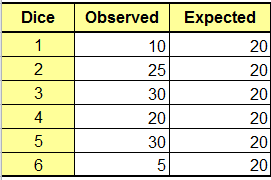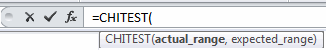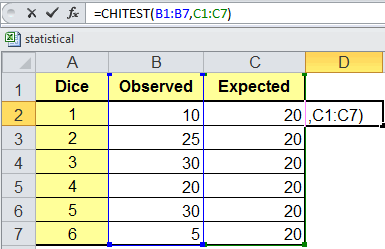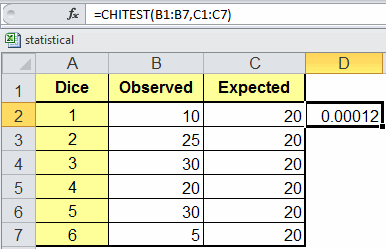# Chi Square Goodness of Fit Test in Excel

There are different types of Chi Square tests:

### Chi Square Goodness of Fit Test

A Chi Square Goodness of Fit test evaluates the probabilities of multiple outcomes.

### Las Vegas Dice Chi Square Goodness of Fit Test Example

Let's say you want to know a six-sided die is fair or unfair (Advanced Statistics by Dr. Larry Stephens). If the die is fair then each side will have an equal probability of coming up; if not, then one or more of the sides will come up more often. Now, test 120 rolls of the die and enter the data into Excel. We would expect each side of the die to come up 20 times (120/6):Then, in an empty cell, begin typing the formula "=chitest(" and Excel will prompt for the observed and expected ranges:Use your mouse to select the Observed (actual_range) and Expected range. Put a comma between the two and a parenthesis at the end and hit return.The Chi Square test will calculate the probability (i.e., p value) of all sides being equal:### Interpreting the Chi Square Goodness of Fit results

• H0: p1 = p2 = p3 = p4 = p5 = p6 = 1/6
• Ha : At least one p is not equal to 1/6
 If Then p value < a Reject the null hypothesis p value > a Cannot Reject the null hypothesis (Accept the null hypothesis)

In the above results the p value = .00012 which is much lower than our alpha value of 0.05, so, we can reject the null hypothesis that the die is fair.

### Why Choose QI Macros Statistical Software for Excel?### Easy to Use

• Works Right in Excel
• Interprets p-values for You
• Accurate No-Worry Results
• Free Training Anytime### Proven and Trusted

• 100,000 Users in 80 Countries
• Celebrating 20th Anniversary
• Five Star CNET Rating - Virus Free### Affordable

• Only \$349 USD
Quantity Discounts Available
• No annual fees
• Free Technical Support